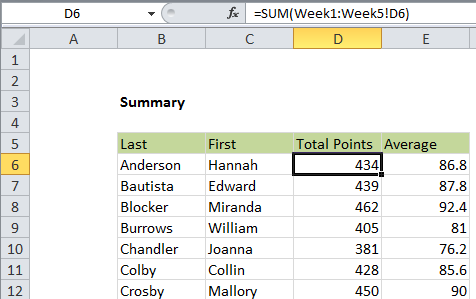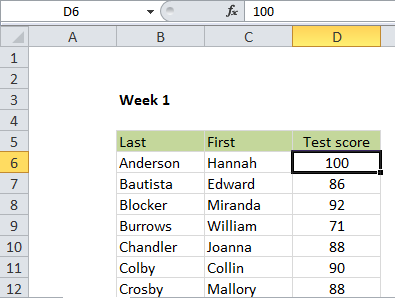## Excel Office

Excel How Tos, Tutorials, Tips & Tricks, Shortcuts

# 3D sum multiple worksheets in Excel

This tutorial shows how to 3D sum multiple worksheets in Excel using the example below;

## Formula

`=SUM(Sheet1:Sheet3!A1)`## Explanation

To sum the same range in one or more sheets, you can use the SUM formula with a special syntax called a “3d reference”.

In the example shown, the formula in D6 is:

`=SUM(Week1:Week5!D6)`

### How this formula works

The syntax for referencing a range of sheets is a built-in feature and works a bit like a reference to a range of cells. For example

`Sheet1:Sheet3!A1`

Means: cell A1 from Sheet1 to Sheet3.

Also See:   How to calculate average last N values in a table in Excel

In the example shown:

`=SUM(Week1:Week5!D6)`

Will sum cell D6 from Week1 to Week5, equivalent to:

`=SUM(Week1!D6,Week2!D6,Week3!D6,Week4!D6,Week5!D6)`

In this workbook, the sheets Week1 through Week 6 all look exactly like this:### Other examples

You can use 3D references in other formulas as well. For example, the formula in E6 averages D6 like this:

`=AVERAGE(Week1:Week5!D6)`
Also See:   Average numbers ignore zero in Excel